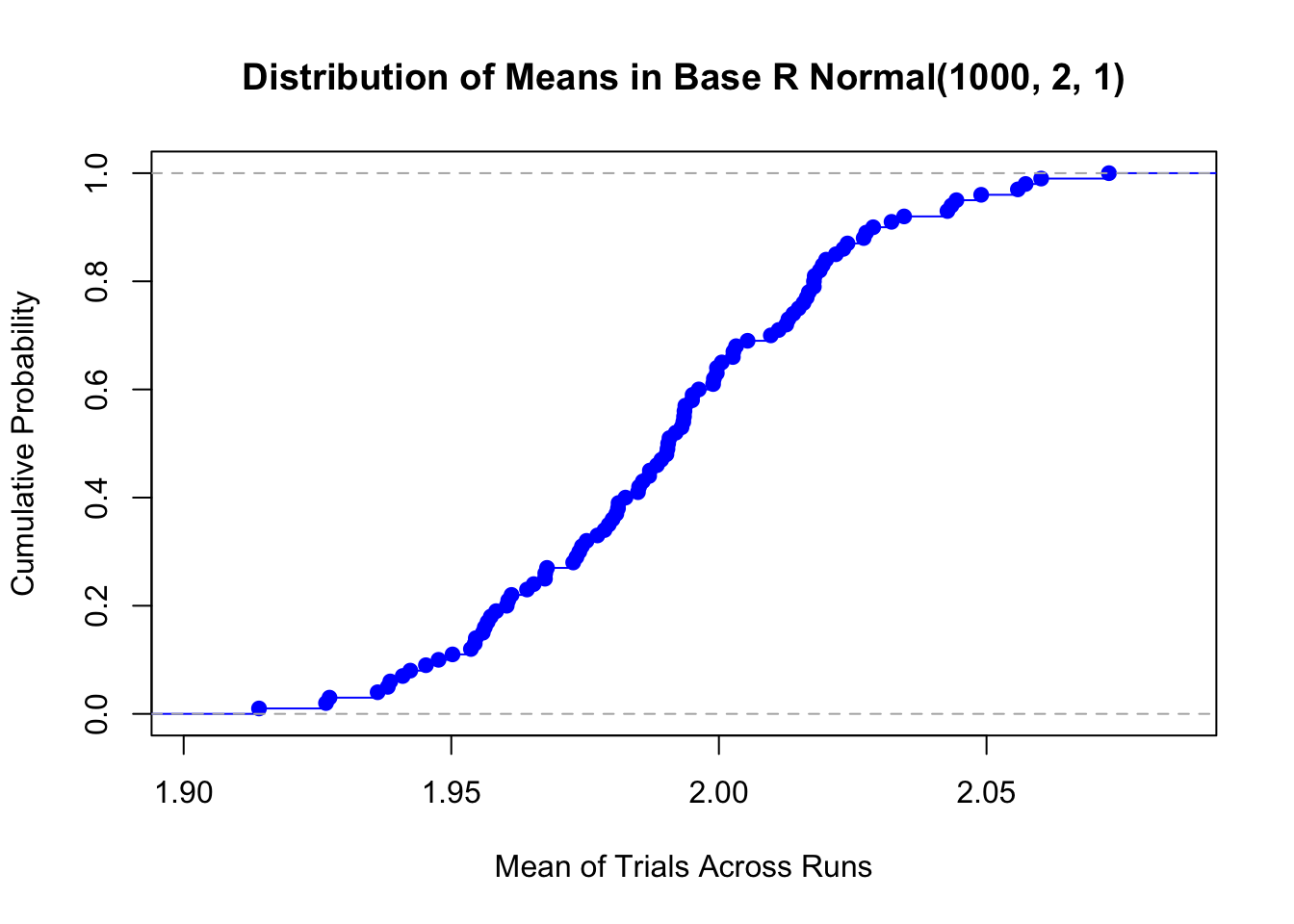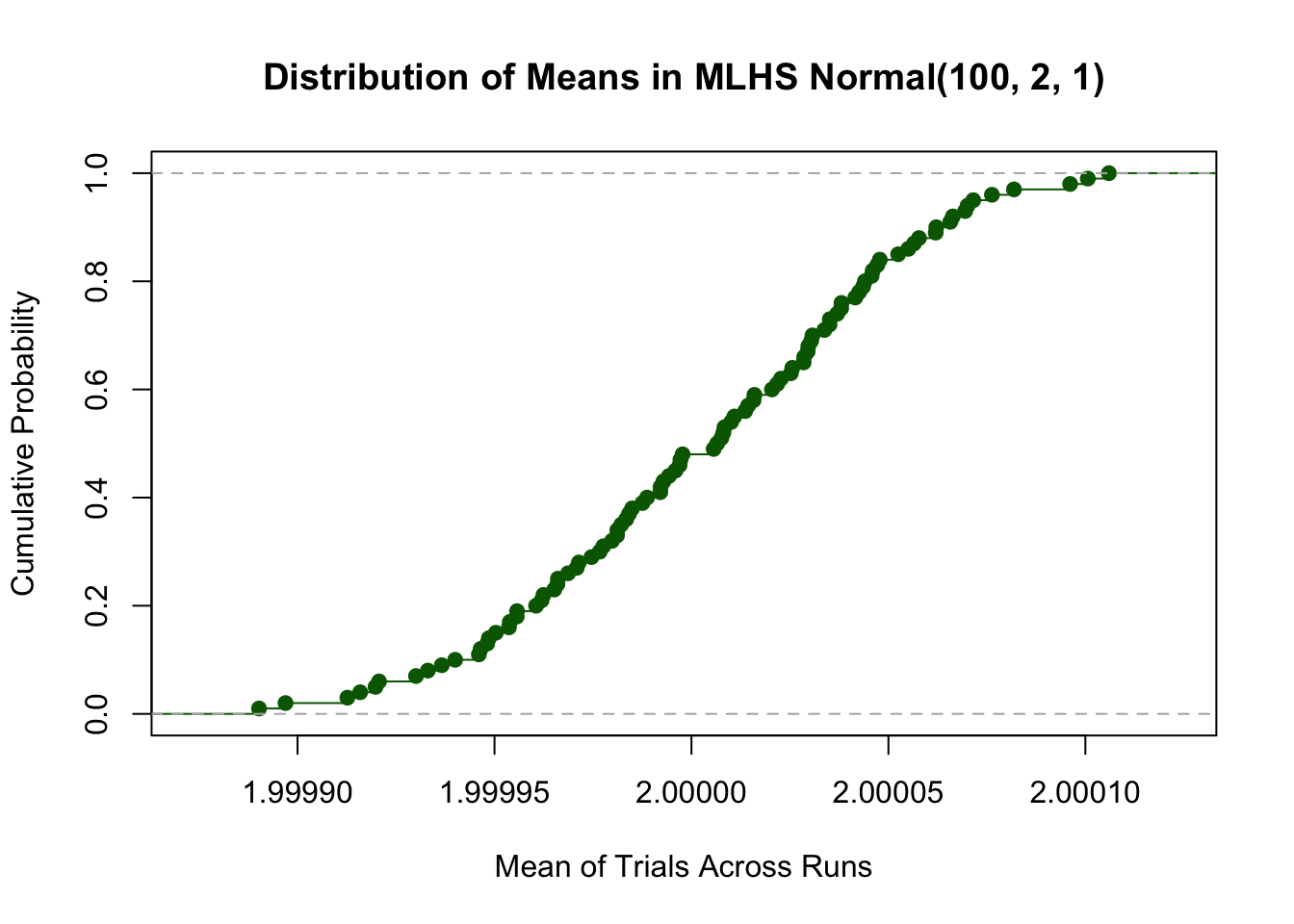## Introduction

I have recently developed two packages that can accompany the modeling simulation platform I described in my book Business Case Analysis with R: Simulation Tutorials to Support Complex Business Decisions (available at Springer-Nature/Apress and Amazon). These packages are:

• leonRdo 0.1.5 : provides median Latin hypercube sampling
• inteRest 1.0 : provides basic finance functions

## Contact

For issues, suggestions, or kudos related to the leonRdo and inteRest R packages, please send me a note at rdbrown@incitedecisiontech.com or visit my Contact web page to send me a note via an easy to use form. I would love to know more about how you might be using these packages.

## leonRdo

The leonRdo package reproduces the standard Monte Carlo distributions using the median Latin hypercube sampling (MLHS) process. This process is used to preserve the theoretical shape and mean of a distribution with fewer samples than required using simple Monte Carlo. Most of the distributions are derived from the base R distributions qxxx. Each distribution returns a vector of values of length equal to the number of indicated samples. As a free-be, this package also provides a means to calculate the cross product of two vectors in R3 space and it provides the Iman-Conover correlation method for imposing correlation to a matrix of independent samples via a target correlation matrix.

The full list of functions available in the package is

• correlate_vars: Iman-Conover Correlation Method
• cross_product: Cross Product of Two Vectors in R3 Space
• rbeta_mlhs: Beta Distribution
• rbinom_mlhs: Binomial Distribution
• rbrwnjn_mlhs: Brown-Johnson Distribution
• rbrwnlog_mlhs: Brown Piecewise Distribution
• rcauchy_mlhs: Cauchy Distribution
• rchisq_mlhs: Chi Square Distribution
• rexp_mlhs: Exponential Distribution
• rgamma_mlhs: Gamma Distribution
• rgeom_mlhs: Geometric Distribution
• rhyper_mlhs: Hypergeometric Distribution
• rlnorm_mlhs: Lognormal Distribution
• rnbinom_mlhs: Negative Binomial Distribution
• rnorm_mlhs: Normal Distribution
• rpois_mlhs: Poisson Distribution
• rtriang_mlhs: Triangular Distribution
• rt_mlhs: Student’s T Distribution
• runif_mlhs: Uniform Distribution
• rweibull_mlhs: Weibull Distribution

### Installation

To install this package, paste the following code in your R console. Don’t forget to specify the ‘lib’ parameter if necessary.

#### For Mac

``````install.packages("https://www.incitedecisiontech.com/packages/leonRdo_0.1.5.tgz",
repos = NULL,
type = "binary")``````

#### For Windows

``````install.packages("https://www.incitedecisiontech.com/packages/leonRdo_0.1.5.tar.gz",
repos = NULL,
type = "source")``````

### Operation

To observe the usefulness of MLHS, consider the distribution of means we obtain by making mutliple independent runs (say, 100) of a Normal distribution (mean = 2, sd = 1) in base R using 1000 trials on each run.

``````runs <- 100
trials <- 1000
test.means.base <- sapply(1:runs, function(r) mean(rnorm(n = trials, 2, 1)))``````Now, observe this distribution of means using MLHS, but this time use just 100 trials.

``````trials <- 100
test.means.mlhs <- sapply(1:runs, function(r) mean(rnorm_mlhs(n = trials, 2, 1)))``````The tradeoff in the much improved stability of the mean of a Normal between trials is the compute-time required to handle the underlying processing of the MLHS. But as we can see, for this example the precision of the base Normal distribution is 684x wider than that of the MLHS while using 10x more trials. To visually reinforce just how stable the MLHS simulation is while using an order of magnitude fewer trials than the base R simulation of the same distribution, consider the scatter plot of the MLHS test means to the base R test means.